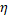# As shown in figure, a liquid of refractive index ‘2’ is poured onto the concave surface of concave – convex lens. R1 and R2 are the radii of curvature of convex and concave surfaces of the lens respectively and R1 = 2R2. The refractive index of material of lens is1. For which combination of1 and2, the whole system behalves as a diverging lens.(a)1 = 1.2 and2 = 1.8 (b)1 = 1.63 and2 = 1.35 (c)1 = 1.56 and2 = 1.33 (d)1 = 1.7 and2 = 1.33

## Question ID - 154040 :- As shown in figure, a liquid of refractive index ‘2’ is poured onto the concave surface of concave – convex lens. R1 and R2 are the radii of curvature of convex and concave surfaces of the lens respectively and R1 = 2R2. The refractive index of material of lens is1. For which combination of1 and2, the whole system behalves as a diverging lens.(a)1 = 1.2 and2 = 1.8 (b)1 = 1.63 and2 = 1.35 (c)1 = 1.56 and2 = 1.33 (d)1 = 1.7 and2 = 1.33

35371 = 1.7 and2 = 1.33

Next Question :
 A convex lens forms a real image of a point object placed on its principal axis. If the upper half of the lens is cut (a)will be shifted downward (b)will be shifted upward (c)(d)